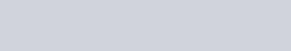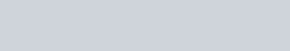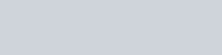HomeQuantitative TechniquesTime, Speed and Distance: A Common CLAT Maths Topic

Time, Speed and Distance: A Common CLAT Maths Topic

Introduction

Time Speed and Distance is one of the most common questions in the CLAT maths papers, which makes it of the most important topics. The questions aren’t tough and a quick read coupled with a little practice will help the aspirants considerably.

Basic Formulae :

1. Speed = Distance/Time
2. Distance = Speed * Time
3. Time = Distance/Speed

Average SpeedAverage Speed when time is constantfor two speeds,Average Speed when the distance is constantfor two speeds,Relative Speed

When travelling in the same direction – (x-y) km/hr

When traveling in opposite direction – (x+y)km/hr

Conversion

km/hr to m/sec – Multiply speed by 5/18

m/sec to km/hr – Multiply speed by 18/5

Illustrations – 1

The distance between two cities Chennai and Trichy is 390 km. Two race bikes Yamaha and Honda simultaneously start from both places and travel towards each other.

The ratio of their speed is 5:8 and the speed of Honda is 80 km/hr. At what time will the bikes meet each other?

Solution :

Let the speed of Yamaha and Honda be 5x,8x respectively.

Hence speed of Yamaha = 5x =50.

So relative speed of 2 bikes = 80+50 = 130.

Meeting time = Total distance/Relative speed = 390/130 = 3 hrs.

Illustration – 2

To cross a poll, a passenger train of 140 metres long, takes 20 seconds. What is the speed of the train?

Solution :

Speed of the train = distance/time = 140/20 = 7 m/sec.

NOTE: Practice as many questions as you can, the key to success in CLAT is scoring in the mathematics paper since it consumes the least time if you’ve put in the hours.

Deal with various types of questions and devote a minimum of 1 hour a day to this segment, this is where most candidates lose marks. All the very best.

Read the post on Clatapult which deals with the same topic here.

Revised and updated on September 4, 2021.